• python列表平均值函数Mathematics and programming go hand in hand. If you are a programmer, at some point you will have to use mathematics. 数学和程序设计齐头并进。 如果您是程序员，则有时必须使用数学。 ...
python列表平均值函数Mathematics and programming go hand in hand. If you are a programmer, at some point you will have to use mathematics.  数学和程序设计齐头并进。 如果您是程序员，则有时必须使用数学。
Data science, machine learning, artificial intelligence, and cryptocurrencies are all based on complex underlying mathematical principles.  数据科学，机器学习，人工智能和加密货币均基于复杂的基础数学原理。
However, using math functions doesn't have to be complex! Python abstracts everything away, so once you understand the concepts, you will not need to understand the full details of the implementation. 但是，使用数学函数不必太复杂！ Python将所有内容抽象化，因此，一旦您理解了这些概念，就无需了解实现的全部细节。
数学不必太吓人 (Math doesn't have to be scary)
There are a lot of mathematical functions you will come across. If you are working with data or analytics, it's important that you understand some mathematical principles and functions.  您将遇到很多数学函数。 如果您正在使用数据或分析，那么了解一些数学原理和函数很重要。
One such function you must understand is the mean function. 您必须了解的一种这样的功能是mean功能。
Don't be put off by the name – there is nothing mean (pun intended) about the mean function in Python.  不要被名字拖延– Python中的mean函数没有任何意义(双关语)。
This post is self contained, but I expect you have some experience working with Python and that you know what a Python list is. If not, check out this article before moving on.  这篇文章是独立的，但是我希望您有使用Python的经验，并且知道Python列表是什么。 如果不是这样，请先阅读本文，然后再继续。
Once you're finished, come back and join me for a deep dive into the mean function. 完成后，请返回并加入我，深入研究mean函数。
统计 (Statistics )
So you want to learn about the mean function. That's great! But before we look at this function its important to look at the discipline from which it originates: statistics.  因此，您想了解mean函数。 那很棒！ 但是，在我们研究此功能之前，先了解其起源的学科很重要：统计学。
In the image above we see a graph. A graph is a pictorial representation that shows the relationship of one variable in relation to another.  在上图中，我们看到了一个图形。 图形是一种图形表示形式，显示了一个变量与另一个变量之间的关系。
Graphs are useful because they allows us to organize data so that we can quickly see trends and relationships between the data. A graph is just one tool that we can use to visualize and analyze data.  图形之所以有用，是因为它们使我们能够组织数据，以便我们可以快速查看数据之间的趋势和关系。 图形只是我们可以用来可视化和分析数据的一种工具。
Statistics is a branch of mathematics that allows us to have a systematic way of classifying, analyzing and interpreting data. This is important because with statistics, we have a collection of ready made tools to do each of those things.  统计学是数学的一个分支，它使我们能够有系统地分类，分析和解释数据。 这一点很重要，因为有了统计信息，我们就可以使用现成的工具来完成所有这些事情。
Imagine if you needed to reinvent a saw every time you needed to cut a piece of wood. We would many people calling saws by different names, even though they do the same thing. To avoid this problem, we gave the saw a name that everyone can refer to it by.  想象一下，如果您每次需要切割一块木头时都需要重新制作锯。 即使他们做同样的事情，我们也会有许多人用不同的名字称锯。 为避免出现此问题，我们给了锯一个名称，每个人都可以引用它。
The same happens in statistics — we have tools well known tools that everyone is familiar with. One such tool is the mean. 统计数据中也是如此-我们拥有大家都熟悉的众所周知的工具。 平均值就是这样一种工具。
模式，中位数和均值 (Mode, Median and Mean)
Though mean is perfectly capable of standing on its own, it's usually taught as part of a trio, which includes the mode, median, and mean.  尽管平均数完全有能力独立存在，但通常将其作为三重奏的一部分进行讲授，包括模式，中位数和均值。
Let's look at a group of numbers so you'll understand what is happening here. Imagine you have the numbers below: 让我们看一组数字，以便您了解这里发生的情况。 假设您有以下数字：
1，2，3，3，4，6，9 (1, 2, 3, 3, 4, 6, 9)
Say we wanted to express which number occurs the most times. It would be 3, and the name we give this property is mode. The mode is the number which is the most frequent in a set we are examining. 假设我们想表达哪个数字出现次数最多。 它将是3，而我们给此属性的名称是mode。 模式是我们正在检查的集合中最频繁的数字。
The number in the middle of an ordered set is called the median. To find the median of a numerical set, arrange the numbers from smallest to largest and then look at the number in the middle. The set of numbers above is already arranged from least to greatest, so the median number is also 3. 有序集合中间的数字称为中位数。 要查找数值集的中位数，请从最小到最大排列数字，然后查看中间的数字。 上面的数字集已经按照从最小到最大的顺序排列，因此中位数也是3。
Finally, the mean is another way to refer to the average of the set. To find the mean, just add all the numbers together and divide it by the total number of elements in the set. In the case of the numbers above, if we add them all together, we get 28. The total number of items in the set is  7, so our mean is 4.  最后，均值是引用集合平均值的另一种方法。 要找到均值，只需将所有数字加在一起，然后除以集合中元素的总数即可。 在上述数字的情况下，如果将它们全部加在一起，我们将得到28。集合中的项目总数为7，因此我们的均值为4。
为什么我们需要中庸？ (Why Do We Need the Mean?)
So at this point you may be wondering why would we need to find the mean of a number anyway.  因此，在这一点上，您可能想知道为什么我们仍然需要找到数字的均值。
The thing is, even statistics itself is subdivided into several groups. Just as you have tools that are used for working with wood and others for working with metal, some tools in statistics are grouped into classes since they are used for a similar purpose.  事实是，甚至统计本身也被细分为几组。 就像您拥有用于木材加工的工具和其他用于金属加工的工具一样，统计中的某些工具也归类为类，因为它们用于类似目的。
One such group in statistics is called summary statistics. One of the things statistics is used for is to describe data, and summary statistics is a collection of tools used for that purpose. One of the items in that class of tools is the mean. 统计中的一种这样的组称为汇总统计。 统计信息的用途之一是描述数据，摘要统计信息是用于此目的的工具的集合。 该类工具中的一项是平均值。
The mean is important due to helping us analyze what is known as a distribution. In statistics, a distribution is a method we use to look at a variable we want information on. Using a distribution we will look at the values of this variable and how often it occurs.  由于有助于我们分析所谓的分布，因此平均值很重要。 在统计数据中，分布是一种用于查看需要信息的变量的方法。 使用分布，我们将查看此变量的值及其发生的频率。
If we collect data, a common type of distribution we see is the normal distribution which takes the form of the bell curve: 如果我们收集数据，我们会看到一种常见的分布类型，它是呈钟形曲线形式的正态分布：
That is to say the variable will have a common value toward which it tends, as well as a starting point and an end point.  也就是说，变量将具有其趋向于的共同值，以及起点和终点。
What the mean does is that it allows us to take a distribution like this and look at the central tendency of the variable, which is the point at which the values of the variable tend to cluster.  平均值的意思是，它允许我们采用这样的分布并查看变量的集中趋势，即变量值趋于聚集的点。
Thus we can say the mean describes the central tendency of the distribution.  因此，我们可以说均值描述了分布的集中趋势。
用Python计算平均值 (Calculating the Mean in Python )
We can manually calculate the mean if we have a small numerical data set it we have a few values to work with. However, when we have hundreds or thousands of values in a data set it becomes impossible to calculate it by hand. 如果我们有一个小的数值数据集，我们可以手动计算平均值，但我们需要处理一些值。 但是，当我们在一个数据集中有成百上千个值时，就无法手动计算它。
Since Python is a "batteries included" language, the way we can do this is to use the mean function of the statistics module within Python.  由于Python是一种“包含电池”的语言，因此，我们可以使用Python中统计模块的mean函数来实现。
Let's use the mean function to calculate the mean of the numerical data set we had earlier in the post: 让我们使用mean函数来计算我们之前发布的数值数据集的均值：
# 1. import the statistics module
import statistics

# 2. list containing our numerical data set
numerical_data_set = [1, 2, 3, 3, 4, 6, 9]

# 3. calculate the mean
calc_mean = statistics.mean(numerical_data_set)

# 4. print our calculated mean
print("Mean is: ", calc_mean)Our code consists of a 4 step sequence that we can use to calculate the mean: 我们的代码由4个步骤组成，可用于计算均值：
We import the statistics module that contains our mean function 我们导入包含平均值函数的统计模块 We create a Python list containing the numerical data set of which we would like to calculate the mean 我们创建一个Python列表，其中包含我们想要计算均值的数值数据集 We calculate the mean and store the result in a variable, calc_mean 我们计算平均值并将结果存储在变量calc_mean We output our calculated mean so that we can get visual feedback 我们输出计算出的平均值，以便获得视觉反馈 When we run the code, we will get the following output: 运行代码时，将获得以下输出：
The program outputs the same value as our manual calculations. When we are working with large data sets, this function will be able to scale to handle whatever we can throw at it.  该程序输出与我们的手动计算相同的值。 当我们处理大型数据集时，此函数将能够扩展以处理我们可以扔给它的任何东西。
结语 (Wrapping Up)
In this post we looked at the mean function in Python. We began by discussing statistics as a whole, then took a deep dive into mean. 在这篇文章中，我们研究了Python中的mean函数。 我们从讨论整个统计数据开始，然后深入探讨均值。
Now that you have a solid understanding of statistics and the mean function in Python, you can use it in your own programs. 既然您对Python的统计数据和mean函数有了深入的了解，就可以在自己的程序中使用它。
If you liked this article, then you may also be curious about learning about data structures and algorithms. If you want a simple, clear, step by step guide to learning about data structures and algorithms without having to write a single line of code, then you can check out the book Codeless Data Structures and Algorithms.  如果您喜欢本文，那么您也可能对学习数据结构和算法感到好奇。 如果您想要一个简单，清晰，分步的指南来学习数据结构和算法，而不必编写任何代码，那么可以参考《无代码数据结构和算法》一书。
Read the book here: 在这里阅读这本书：
翻译自: https://www.freecodecamp.org/news/how-to-calculate-the-average-of-a-list-statistics-and-pythons-mean-function-explained-in-detail/python列表平均值函数
展开全文• 本文实例讲述了python计算一个序列的平均值的方法。分享给大家供大家参考。具体如下： def average(seq, total=0.0): num = 0 for item in seq: total += item num += 1 return total / num 如果序列是数组...
• ## python求平均值

万次阅读 2019-12-27 20:49:59
python平均值 首先我们先来了解一下计算平均IPO模式. 输入：待输入计算平均数。 处理：平均数算法 输出：平均数 推荐：《python教程》 明白了程序的IPO模式之后，我们打开本地的python的IDE工具，并新建一...
python求平均值
首先我们先来了解一下计算平均数的IPO模式.
输入：待输入计算平均数的数。
处理：平均数算法
输出：平均数
推荐：《python教程》
明白了程序的IPO模式之后，我们打开本地的python的IDE工具，并新建一个python文件，命名为test6.py.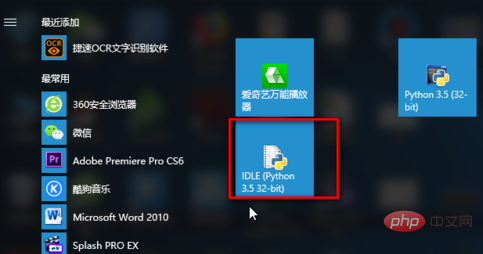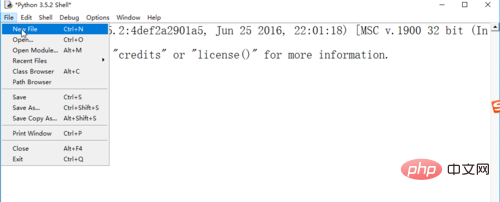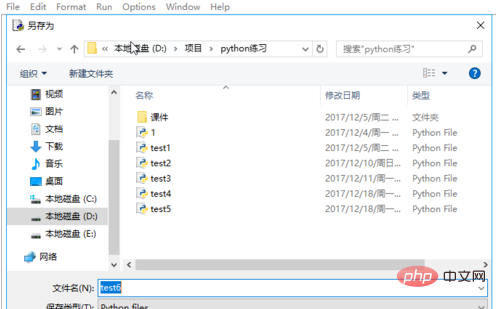打开test6.py,进行编码，第一步，提示用户输入要计算多少个数的平均数。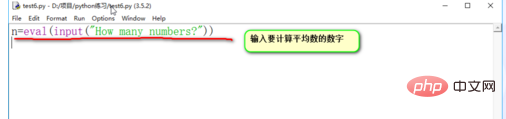第二步，初始化sum总和的值。注意，这是编码的好习惯，在定义一个变量的时候，给一个初始值。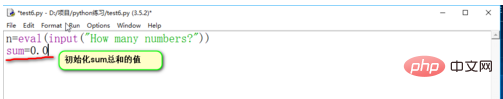第三步，循环输入要计算平均数的数，并计算总和sum的值。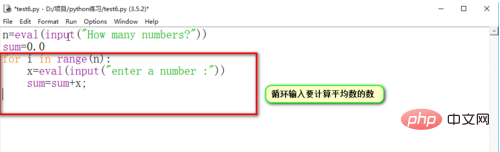最后，计算出平均数，并输出，利用“总和/数量”的公式计算出平均数。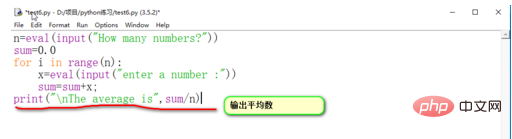编码完成后，记得保存，然后进行调试运行。按F5键或者点击菜单栏中的“run”-》“run model”来运行程序。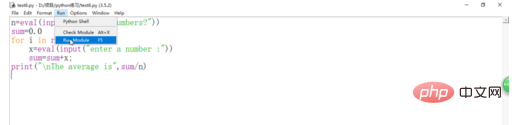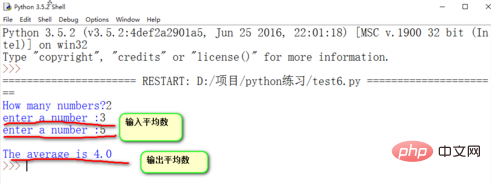相关文章推荐：
1.如何用python求平均值
2.python中平均分割列表
相关视频推荐：
1.小甲鱼零基础入门学习Python视频教程
2.python交流群资料分享：887934385
以上就是python求平均值的详细内容，更多请关注请继续关注公众号python社区营其它相关文章！


展开全文• 编写主程序，输入列表，调用函数计算最大、最小和平均值，在主程序中显示它们。注，不能使用Python及第三方库函数直接求。 代码： ''' 编写函数，计算列表最大、最小和平均值。编写主程序，输入列表， 调用函数...
题目要求：

编写函数，计算列表的最大、最小和平均值。编写主程序，输入列表，调用函数计算最大、最小和平均值，在主程序中显示它们。注，不能使用Python及第三方库的函数直接求。

代码：
'''
编写函数，计算列表的最大、最小和平均值。编写主程序，输入列表，
调用函数计算最大、最小和平均值，
在主程序中显示它们。注，不能使用Python及第三方库的函数直接求
written by tyb
2020/10/20
references:
https://blog.csdn.net/u013595395/article/details/88425996
'''
def inputList():
#num_list = list(map(int, input().split()))  # 或者
print("请输入整数，以空格隔开")
num_list = [int(i) for i in input().split()] # 接收一段以空格隔开的整数的输入
return num_list
def outputList(list):
for i in range(len(list)):
print(list[i])
def findMax(list):
num_max = list #记录最大值
for i in range(len(list)):
if list[i] >= num_max:
num_max = list[i]
return num_max
def findMin(list):
num_min = list #记录最小值
for i in range(len(list)):
if list[i] <= num_min:
num_min = list[i]
return num_min
def averageValue(list):
value = 0 #记录平均值
sum = 0 #求和
for i in range(len(list)):
sum = sum + list[i]
value = sum/len(list)
return value

if __name__ == '__main__':
list = inputList()
max = findMax(list)
min = findMin(list)
value = averageValue(list)
print("最大值为：",max)
print("最小值为：",min)
print("平均值为：",value)
#outputList(list)



展开全文1024程序员节 最大值 最小值
• 计算每张图像处理前后的psnr、ssim，然后进行除以总数，分别得到psnr和ssim均值。 只需要修改路径进行指定文件夹的循环计算即可。 import os ...#当中是你的程序 def psnr(img1, img2): ...
计算每张图像处理前后的psnr、ssim，然后进行除以总数，分别得到psnr和ssim均值。

只需要修改路径进行指定文件夹的循环计算即可。

import os
import numpy as np
import math
import cv2

import time

start = time.clock()

#当中是你的程序

def psnr(img1, img2):
mse = np.mean((img1/1. - img2/1.) ** 2 )
if mse < 1.0e-10:
return 100*1.0
return 10 * math.log10(255.0*255.0/mse)

def mse(img1,img2):
mse = np.mean((img1/1. - img2/1.) ** 2 )
return mse

def ssim(y_true , y_pred):
u_true = np.mean(y_true)
u_pred = np.mean(y_pred)
var_true = np.var(y_true)
var_pred = np.var(y_pred)
std_true = np.sqrt(var_true)
std_pred = np.sqrt(var_pred)
c1 = np.square(0.01*7)
c2 = np.square(0.03*7)
ssim = (2 * u_true * u_pred + c1) * (2 * std_pred * std_true + c2)
denom = (u_true ** 2 + u_pred ** 2 + c1) * (var_pred + var_true + c2)
return ssim / denom

path1 = 'D:/sy_imags1000_80lun/outputs/'  #指定输出结果文件夹
path2 = 'D:/sy_imags1000_80lun/targets/'#指定原图文件夹
f_nums = len(os.listdir(path1))
list_psnr = []
list_ssim = []
list_mse = []
for i in range(1,f_nums+1):
img_a = cv2.imread(path1+str(i)+'-outputs.png')
img_b = cv2.imread(path2+str(i)+'-targets.png')
psnr_num = psnr(img_a, img_b)
ssim_num = ssim(img_a, img_b)
mse_num = mse(img_a,img_b)
list_ssim.append(ssim_num)
list_psnr.append(psnr_num)
list_mse.append(mse_num)
print("平均PSNR:",np.mean(list_psnr))#,list_psnr)
print("平均SSIM:",np.mean(list_ssim))#,list_ssim)
print("平均MSE:",np.mean(list_mse))#,list_mse)

elapsed = (time.clock() - start)
print("Time used:",elapsed)


展开全文• 学习了Python相关数据类型，函数的知识后，利用字符串的分割实现了输入任意多个数据，并计算平均值的程序。思路是接收输入的字符串，以空格为分隔符，将分割的数据存入列表（lst1）中，将lst1中的数据转存入另一...
• 下面我们用协程来实现平均值的计算，区别之前的闭包。 这个无限循环表明，只要调用方不断把值发给这个协程，它就会一直接收值，然后生成 结果。仅当调用方在协程上调用 .close() 方法，或者没有对协程的引用而被...
• 学习了Python相关数据类型，函数的知识后，利用字符串的分割实现了输入任意多个数据，并计算平均值的程序。思路是接收输入的字符串，以空格为分隔符，将分割的数据存入列表（lst1）中，将lst1中的数据转存入另一...
• #!/usr/bin/python # -*- coding: UTF-8 -*- #指定编码格式，让python文件...#判断输入学生数是否超出这个范围避免int(4字节)无法放下max导致产生随机数 while True: student_number = int(input("请输入班级py 学习 练习
• 代码如下： 1 import random 2 3 n = 0 4 sum = 0 5 while n < 10: 6 num = random.randint(1, 100) 7 sum = sum + num ...12 print("10个数的平均值为:%.2f" % (sum / 10)) 运行结pytho
• 利用字符串的分割实现了输入任意多个数据，并计算平均值的程序。思路是接收输入的字符串，以空格为分隔符，将分割的数据存入列表（lst1）中，将lst1中的数据转存入另一个空列表（lst）中，转存时将字符串转化为...string 笔记
• 这里用到了itertools模块中permutations(实质上就是排列功能)，当然itertools模块中还有combinations(实质上就是组合功能)，且看程序。 from itertools import permutations li_per = list(permutations("...
• 获取以逗号分隔多个数据输入（输入为一行），计算基本统计值（平均值、标准差、中位数）。除中位数外，其他输出保留小数点后两位。‪‬‪‬‪‬‪‬‪‬‮‬‫‬‫‬‪‬‪‬‪‬‪‬‪‬‮‬‪‬‭‬‪‬‪‬‪‬...
• 基本统计值计算 这一一个计算多数据基本统计...输出：平均值，方差，中位数 from math import sqrt def getNum(): nums = [] iNumStr = input("请输入数字(直接输入回车退出):") while iNumStr != "": nums.appe
• 使用OpenCV构建图像识别算法，识别图片中米粒个数，并计算米粒的平均面积和长度 软件架构 模块：OpenCV 4.0.0.21 编程语言：Python 3.7.2 编译器：PyCharm 2018 程序设计思路 首先介绍一下程序设计思路： ...
• ema其公式为：（[[或参考百度百科](https://baike.baidu.com/item/EMA/12646151?fr=aladdin "")](https://baike.baidu.com/item/EMA/12646151?fr=aladdin "")） ... 此处计算a移动平均值ema print(ema)macd
• 1054. 求平均值 (20) 时间限制 400 ms ...判题程序 ...本题基本要求非常简单：给定N个实数，计算它们的平均值。但复杂是有些输入数据可能是非法。一个“合法”输入是[-1000，1000]区间PAT
• 在 Wikipedia 上搜索 UCI ProTour 自行车手统计数据（身高、体重、国家/地区、年龄）以计算平均值。 用法 重建数据库 要重建数据库，只需运行python ProTrawler.py 。 这会将data.js输出到当前工作目录。 重建网络...JavaScript
• 要求进行如下统计：所有人的应发工资总额、所有人的扣除工资总额、所有人的实发工资总额，及上述三个统计值的平均值。输出实发工资最高、最低的员工工资条 **其中加了几条数据，但是运行不出来** ` print...
• Python】第4次练习：for循环、while循环 一、单选题： 二、程序题： 1、字符串垂直输出 x=input() for i in x: print(i) 2、N多次方 N=eval(input()) for i in range(6): if i==5: ...for循环 while循环
• 总个数、求和、平均值、方差、中位数… 如： - 总个数：len() - 求和：for … in - 平均值：求和/总个数 - 方差：各数据与平均数差平方和平均数 - 中位数：排序，然后… 奇数找中间1个，偶数找中间2个取...
• 标题python编程：学校举办新生歌手大赛，每个选手成绩由评委评分去掉一个最高分和一个最低分剩下的平均值得到。编写程序实现：第一行输入一个数字代表评委个数n，从第二行开始每行输入一个评委得分（共n行）...
• 2.4.1 求和、平均值、方差.................54 2.4.2 最值和排序.................................55 2.4.3 多项式函数.................................57 2.4.4 分段函数........................................
• 利用二维列表建立一个4*4矩阵。要求每个元素是一个利用random库建立[20，50]间随机整数。计算该矩阵每一行的平均值和每一列的平均值，分别形成平均值列表和列平均值列表，并输出。</p>有问必答
• 计算三个数字的平均值 使用单个变量计算器 使用Selenium进行自动化以填写表格并将输出发送到Telegram中聊天室 使用Python，OpenCV进行人群控制并将数据保存在InfluxDB中 该脚本以每分钟#ToTheMoon狗狗币价格...
• 输入固定参数，就能得到预测和控制范围，其中有数值需要一定手算来输入，比方说平均值什么，只要是为了写作业用，考试时候也需要计算这些东西，程序代替都是些重复步骤，有兴趣可以自己扩展与延申
• 最好有几种计算相同日照值的方法来测试输出日照的方法依赖性。 目前，唯一可用的方法是基于 Laskar2004 的 fortran 程序： : 另一个新增功能是用于对日照输出进行统计分析的功能。 我正在考虑将日照的功率谱时间...
• 多做2个重复取平均值。然后采用灰度分析进行内参调整，从而调整之后上样量。 自变量与因变量一系列对应数据,(x1,y1),(x2,y2),(x3,y3),...(xn,yn)，分别是已经做好实验蛋白质浓度和相对应灰度值。Input，以......

# python计算平均值的程序python 订阅